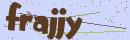# TP 或者 Laravel 框架实现无限级分类

```public function get_all_data()
{
//TP框架查询所有数据：
//\$data = (new UserModel)->select();
//Laravel 框架查询所有数据：
\$data = (new UserModel)->get();
\$res = \$this->sort_data(\$data);
dump(\$res);
}

public function sort_data(\$data, \$pid = 0, \$root = 1)
{
static \$new_data = [];
//循环遍历传递过来的数据
foreach (\$data as \$key => \$value) {
//判断数据的上级 ID 是否等于当前 ID，最顶级 ID 为 0
//这里的上级 ID 在数据库中的字段为 parent_id
if (\$value['parent_id'] == \$pid) {
\$new_data[] = \$value;
\$value['root'] = \$root;
//使用当前数据记录的 ID 进行再次循环排序
\$this->sort_data(\$data, \$value['id'], \$root + 1);
}
}
return \$new_data;
}```

```[{
title: '一级菜单',
children: [{
title: '二级菜单',
children: [{
title: '三级菜单'
//……
//以此类推, 无限层级
}]
}]```

```public function sort_data(\$data, \$pk = 'id', \$pid = 'pid', \$child = 'children', \$root = 0)
{
// 创建Tree
\$tree = [];
if (!is_array(\$data)) {
return false;
}

//创建基于主键的数组引用
\$refer = [];
foreach (\$data as \$key => \$value_data) {
\$refer[\$value_data[\$pk]] = &\$data[\$key];
}
foreach (\$data as \$key => \$value_data) {
// 判断是否存在parent
\$parentId = \$value_data[\$pid];
if (\$root == \$parentId) {
\$tree[] = &\$data[\$key];
} else {
if (isset(\$refer[\$parentId])) {
\$parent = &\$refer[\$parentId];
\$parent[\$child][] = &\$data[\$key];
}
}
}

return \$tree;
}```

```public function get_all_data()
{
//TP 框架查询所有数据：
//\$data = (new UserModel)->select();
//Laravel 框架查询所有数据：
\$data = (new UserModel)->get();

foreach (\$data as \$key => \$value) {
unset(\$data[\$key]['content']);
unset(\$data[\$key]['list']);
//... 以此类推
}

\$res = \$this->sort_data(\$data);
dump(\$res);
}```

```public function get_all_data()
{
//TP框架查询所有数据：
//\$data = (new UserModel)->select();
//Laravel 框架查询所有数据：
\$data = (new UserModel)->get();

\$new_data = [];
foreach (\$data as \$key => \$value) {
\$new_data[\$key]['id'] = \$value['id'];
\$new_data[\$key]['pid'] = \$value['pid'];
\$new_data[\$key]['title'] = \$value['title'];

//... 以此类推
//此方法一定要将 id 和 pid 也存入新数组，否则无法实现无限级。
}

\$res = \$this->sort_data(\$data);
dump(\$res);
}```